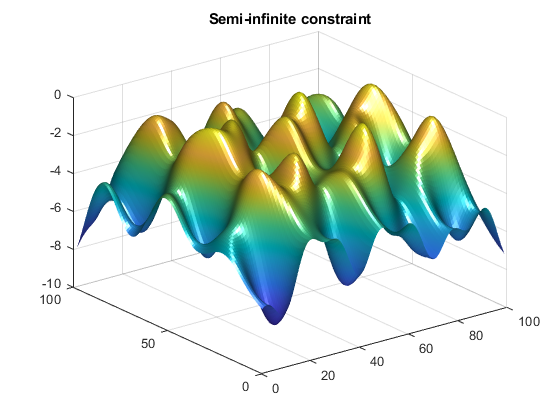Documentation

Two-Dimensional Semi-Infinite Constraint

Find values of x that minimize

f(x) = (x1 – 0.2)2 + (x2– 0.2)2 + (x3– 0.2)2,

where

for all values of w1 and w2 over the ranges

1 ≤ w1 ≤ 100,
1 ≤ w2 ≤ 100,

starting at the point x = [0.25,0.25,0.25].

Note that the semi-infinite constraint is two-dimensional, that is, a matrix.

First, write a file that computes the objective function.

function f = myfun(x,s)
% Objective function
f = sum((x-0.2).^2);

Second, write a file for the constraints, called mycon.m. Include code to draw the surface plot of the semi-infinite constraint each time mycon is called. This enables you to see how the constraint changes as X is being minimized.

function [c,ceq,K1,s] = mycon(X,s)
% Initial sampling interval
if isnan(s(1,1)),
s = [2 2];
end

% Sampling set
w1x = 1:s(1,1):100;
w1y = 1:s(1,2):100;
[wx,wy] = meshgrid(w1x,w1y);

% Semi-infinite constraint
K1 = sin(wx*X(1)).*cos(wx*X(2))-1/1000*(wx-50).^2 -...
sin(wx*X(3))-X(3)+sin(wy*X(2)).*cos(wx*X(1))-...
1/1000*(wy-50).^2-sin(wy*X(3))-X(3)-1.5;

% No finite nonlinear constraints
c = []; ceq=[];

% Mesh plot
m = surf(wx,wy,K1,'edgecolor','none','facecolor','interp');
title('Semi-infinite constraint')
drawnow

Next, invoke an optimization routine.

x0 = [0.25, 0.25, 0.25];    % Starting guess
[x,fval] = fseminf(@myfun,x0,1,@mycon)

After nine iterations, the solution is

x
x =
0.2523    0.1715    0.1938

and the function value at the solution is

fval
fval =
0.0036

The goal was to minimize the objective f(x) such that the semi-infinite constraint satisfied K1(x,w) ≤ 1.5. Evaluating mycon at the solution x and looking at the maximum element of the matrix K1 shows the constraint is easily satisfied.

[c,ceq,K1] = mycon(x,[0.5,0.5]);  % Sampling interval 0.5
max(max(K1))

ans =
-0.0370

This call to mycon produces the following surf plot, which shows the semi-infinite constraint at x.